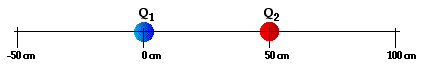# Electricity: Static Electricity

## Static Electricity: Audio Guided Solution

#### Problem 32:

Two point charges (Q1 and Q2) are positioned along the x-axis as shown below.They are spaced 50. cm apart and have charges of +38 nC (Q1) and -18 nC (Q2). Determine the location along the axis where the net electric field is 0 N/C.

## Habits of an Effective Problem Solver

• Read the problem carefully and develop a mental picture of the physical situation. If necessary, sketch a simple diagram of the physical situation to help you visualize it.
• Identify the known and unknown quantities; record them in an organized manner. A diagram is a great place to record such information. Equate given values to the symbols used to represent the corresponding quantity - e.g., Q1 = 2.4 μC; Q2 = 3.8 μC; d = 1.8 m; Felect = ???.
• Use physics formulas and conceptual reasoning to plot a strategy for solving for the unknown quantity.
• Identify the appropriate formula(s) to use.
• Perform substitutions and algebraic manipulations in order to solve for the unknown quantity.